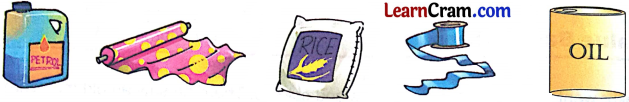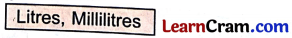# DAV Class 3 Maths Chapter 10 Worksheet 1 Solutions

The DAV Maths Book Class 3 Solutions and DAV Class 3 Maths Chapter 10 Worksheet 1 Solutions of Capacity offer comprehensive answers to textbook questions.

## DAV Class 3 Maths Ch 10 WS 1 Solutions

Solve the following:

Question 1.
9 litres = 9 × _____ ml = _____ ml
Solution:
9 litres = 9 × 1000 ml = 9000 ml

Question 2.
2 litres = 2 × _____ ml = _____ ml
Solution:
2 litres = 2 × 1000 ml = 2000 ml

Question 3.
4 litres = 4 × _____ ml = _____ ml
Solution:
4 litres = 4 × 1000 = 4000 mlQuestion 4.
5 litres = _____ ml
Solution:
5 litres = 5000 ml

Question 5.
18 litres = _____ ml
Solution:
18 litres = 18000 ml

Question 6.
3 litres = _____ ml
Solution:
3 litres = 3000 ml### DAV Class 3 Maths Chapter 10 Worksheet 1 Notes

• 1 litre = 1000 millilitre
• The standard unit of capacity is Litre.
• We write litres as 7’ and millilitres as ‘ml’.
• We multiply the number of litres by 1000 to convert ‘litres’ into ‘millilitres’.

Question 1.
You are given some objects. Encircle all the objects which are sold by measuring capacity.Solution:
Do it yourself.

Question 2.
Compare the following using (>) or (<).

(a) Capacity of a water tank _____ capacity of a bucket.
Solution:
Capacity of a water tank > capacity of a bucket.

(b) Capacity of a glass _____ capacity of a jug.
Solution:
Capacity of a glass < capacity of a jug.

(c) Capacity of ink in your pen _____ capacity of ink in an inkpot.
Solution:
Capacity of ink in your pen < capacity of ink in an inkpot.

(d) Capacity of milk in 1 litre polybag _____ capacity of milk in a 1/2 litre polybag.
Solution:
Capacity of milk in 1 litre polybag > capacity of milk in a 1/2 l polybag.Question 3.
Which unit from the box will you use to measure the following:(a) Milk in a cup ________
Solution:
Millilitres

(b) Oil in a spoon ________
Solution:
Millilitres

(c) Water in a tank ________
Solution:
Litres

(d) Petrol in a car ________
Solution:
Litres(e) Water in a glass ________
Solution:
Millilitres

(f) Ink in your pen ________
Solution:
Millilitres

(g) Water in a bucket ________
Solution:
Litres

(h) Juice in a bottle ________
Solution:
LitresConversions

1 litre = 1000 millilitres
Ex. 1. 4 l = 4 × 1000 millilitres = 4000 ml
Ex. 2. 5l = 5 × 1000 ml = 5000 ml.